Open in App
Not now

# PySpark DataFrame – Select all except one or a set of columns

• Last Updated : 17 Jun, 2021

In this article, we are going to extract all columns except a set of columns or one column from Pyspark dataframe. For this, we will use the select(), drop() functions.

But first, let’s create Dataframe for demonestration.

## Python3

 `# importing module``import` `pyspark`` ` `# importing sparksession from pyspark.sql module``from` `pyspark.sql ``import` `SparkSession`` ` `# creating sparksession and giving an app name``spark ``=` `SparkSession.builder.appName(``'sparkdf'``).getOrCreate()`` ` `# list  of students  data``data ``=` `[[``"1"``, ``"sravan"``, ``"vignan"``],``        ``[``"2"``, ``"ojaswi"``, ``"vvit"``],``        ``[``"3"``, ``"rohith"``, ``"vvit"``],``        ``[``"4"``, ``"sridevi"``, ``"vignan"``],``        ``[``"1"``, ``"sravan"``, ``"vignan"``],``        ``[``"5"``, ``"gnanesh"``, ``"iit"``]]`` ` `# specify column names``columns ``=` `[``'student ID'``, ``'student NAME'``, ``'college'``]`` ` `# creating a dataframe from the lists of data``dataframe ``=` `spark.createDataFrame(data, columns)`` ` `print``(``'Actual data in dataframe'``)``dataframe.show()`

Output: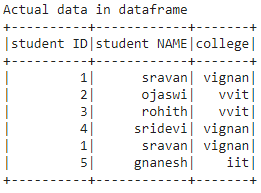### Method 1: Using drop() function

drop() is used to drop the columns from the dataframe.

Syntax: dataframe.drop(‘column_names’)

Where dataframe is the input dataframe and column names are the columns to be dropped

Example: Python program to select data by dropping one column

## Python3

 `# drop student id``dataframe.drop(``'student ID'``).show()`

Output: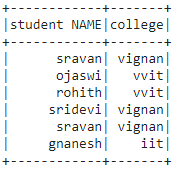Example 2: Python program to drop more than one column(set of columns)

## Python3

 `# drop student id and college``dataframe.drop(``'student ID'``,``'college'``).show()`

Output: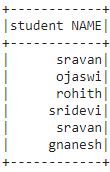### Method 2: Using select() function

This function is used to select the columns from the dataframe

Syntax: dataframe.select(columns)

Where dataframe is the input dataframe and columns are the input columns

Example 1: Select one column from the dataframe.

## Python3

 `# select student id ``dataframe.select(``'student ID'``).show()`

Output: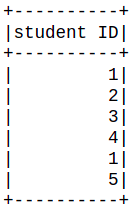Example 2: Python program to select two columns id and name

## Python3

 `# select student id and student name``dataframe.select(``'student ID'``,``'student NAME'``).show()`

Output: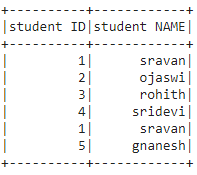My Personal Notes arrow_drop_up